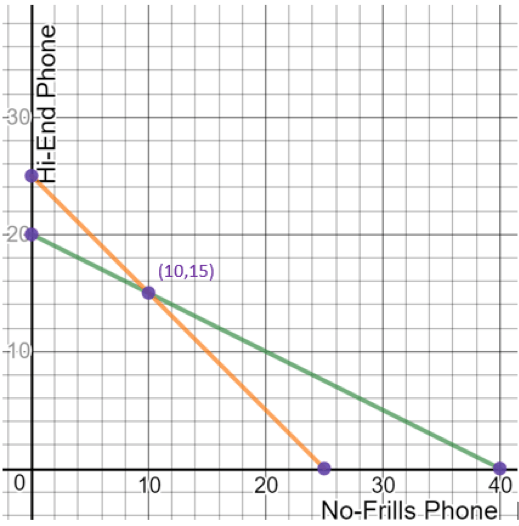# LINR 3 | Lesson 2 | Try This! (Solving Systems of Inequalities)

Let’s step back and look at the systems of equations in the cell phone situation.

Recall, when solving linear systems of equalities, the solution is the point(s) where the two lines intersect. This may be one, none, or infinite.

In the cell phone situation, the solution of the linear equalities would be at the point $$(10,15)$$, where 25 phones are produced in 80 hours. Graphs are created using Desmos.com.Use your own equations to verify that $$(10,15)$$ is a solution.

Did you remember to check both equations?

Now try $$(40,0)$$. What did you find?

Even if the point makes one equation true, in a system, you must verify in both equations.In our cell phone situation, use your inequalities to verify whether or not the following points are solutions:

$$(7,19)\hspace{2mm}(22,3)\hspace{2mm}(20,11)\hspace{2mm}(10,6)\hspace{2mm}(3.5,10)$$

Where can you find the solutions to the cell phone situation in the graph?

In the overlap of green and orange regions, however note that because the cell phones are indivisible physical objects, only the lattice points are possible solutions.

The solution of a system of linear inequalities contains the set of all points which make the given inequalities true. Points in the solution set are located in the shaded regions where the inequalities overlap.

Depending on the situation, the number of solutions to a system of inequalities, can be none, infinite or anything in between.